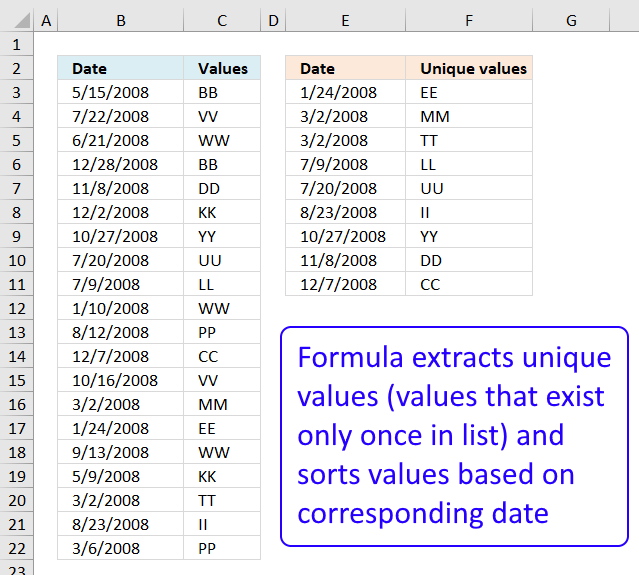Author: Oscar Cronquist Article last updated on November 26, 2018The formula in cell F3 extracts unique values from column C, the formula in cell F3 extracts the corresponding dates from column B.

Formula in E3:

=LOOKUP(2, 1/((COUNTIF(\$C\$3:\$C\$22, \$C\$3:\$C\$22)=1)*(COUNTIF(\$F\$2:F2, \$C\$3:\$C\$22)=0)), \$B\$3:\$B\$22)

copied down as far as necessary.

Array formula in F2:

=LOOKUP(2, 1/((COUNTIF(\$C\$3:\$C\$22, \$C\$3:\$C\$22)=1)*(COUNTIF(\$F\$2:F2, \$C\$3:\$C\$22)=0)), \$C\$3:\$C\$22)

copied down as far as necessary.

### Explaining formula in cell E3

#### Step 1 - Get frequency of each value

The COUNTIF function counts values based on a condition or criteria. We are looking for unique values that is why we compare the array with 1.

COUNTIF(\$C\$3:\$C\$22,\$C\$3:\$C\$22)=1

becomes

COUNTIF({"BB"; "VV"; "WW"; "BB"; "DD"; "KK"; "YY"; "UU"; "LL"; "WW"; "PP"; "CC"; "VV"; "MM"; "EE"; "WW"; "KK"; "TT"; "II"; "PP"}, {"BB"; "VV"; "WW"; "BB"; "DD"; "KK"; "YY"; "UU"; "LL"; "WW"; "PP"; "CC"; "VV"; "MM"; "EE"; "WW"; "KK"; "TT"; "II"; "PP"})=1

becomes

{2;2;3;2;1;2;1;1;1;3;2;1;2;1;1;3;2;1;1;2}=1

and returns

{FALSE; FALSE; FALSE; FALSE; TRUE; FALSE; TRUE; TRUE; TRUE; FALSE; FALSE; TRUE; FALSE; TRUE; TRUE; FALSE; FALSE; TRUE; TRUE; FALSE}

#### Step 2 - Check previous values to prevent duplicate values in output list

COUNTIF(\$F\$2:F2,\$C\$3:\$C\$22)=0

becomes

COUNTIF("Date", {"BB"; "VV"; "WW"; "BB"; "DD"; "KK"; "YY"; "UU"; "LL"; "WW"; "PP"; "CC"; "VV"; "MM"; "EE"; "WW"; "KK"; "TT"; "II"; "PP"})=0

becomes

{0;0;0;0;0;0;0;0;0;0;0;0;0;0;0;0;0;0;0;0}=0

and returns

{TRUE; TRUE; TRUE; TRUE; TRUE; TRUE; TRUE; TRUE; TRUE; TRUE; TRUE; TRUE; TRUE; TRUE; TRUE; TRUE; TRUE; TRUE; TRUE; TRUE}

#### Step 3 - Multiply arrays

We multiply the arrays because both conditions must be met.

((COUNTIF(\$C\$3:\$C\$22,\$C\$3:\$C\$22)=1)*(COUNTIF(\$F\$2:F2,\$C\$3:\$C\$22)=0))

becomes

{FALSE; FALSE; FALSE; FALSE; TRUE; FALSE; TRUE; TRUE; TRUE; FALSE; FALSE; TRUE; FALSE; TRUE; TRUE; FALSE; FALSE; TRUE; TRUE; FALSE}*{TRUE; TRUE; TRUE; TRUE; TRUE; TRUE; TRUE; TRUE; TRUE; TRUE; TRUE; TRUE; TRUE; TRUE; TRUE; TRUE; TRUE; TRUE; TRUE; TRUE}

and returns

{0;0;0;0;1;0;1;1;1;0;0;1;0;1;1;0;0;1;1;0}.

#### Step 4 - Divide 1 with array

This step makes sure that FALSE will become #DIV/0!. The LOOKUP function ignores errors and that will be very useful for us.

1/((COUNTIF(\$C\$3:\$C\$22,\$C\$3:\$C\$22)=1)*(COUNTIF(\$F\$2:F2,\$C\$3:\$C\$22)=0))

becomes

1/{0;0;0;0;1;0;1;1;1;0;0;1;0;1;1;0;0;1;1;0}

and returns

{#DIV/0!; #DIV/0!; #DIV/0!; #DIV/0!; 1; #DIV/0!; 1; 1; 1; #DIV/0!; #DIV/0!; 1; #DIV/0!; 1; 1; #DIV/0!; #DIV/0!; 1; 1; #DIV/0!}

#### Step 5 - Return value

LOOKUP(2,1/((COUNTIF(\$C\$3:\$C\$22,\$C\$3:\$C\$22)=1)*(COUNTIF(\$F\$2:F2,\$C\$3:\$C\$22)=0)),\$B\$3:\$B\$22)

becomes

LOOKUP(2,{#DIV/0!; #DIV/0!; #DIV/0!; #DIV/0!; 1; #DIV/0!; 1; 1; 1; #DIV/0!; #DIV/0!; 1; #DIV/0!; 1; 1; #DIV/0!; #DIV/0!; 1; 1; #DIV/0!},\$B\$3:\$B\$22)

and returns

8/23/2008 in cell E3.

### Download Excel *.xlsx file

Filter unique values and sort by date.xlsx